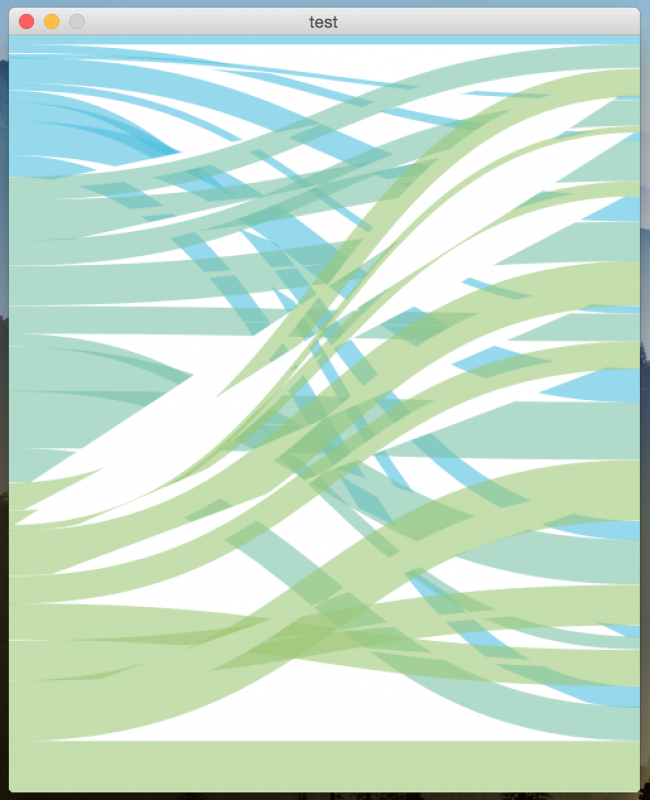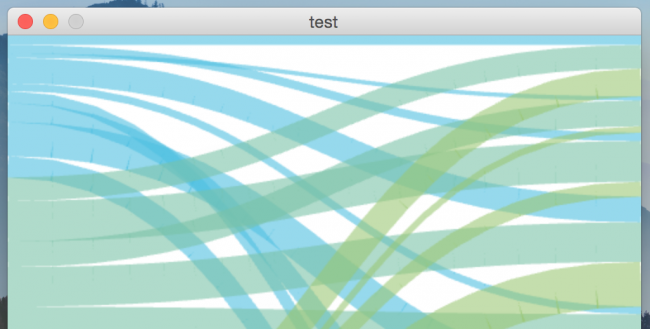#### Howdy, Stranger!

We are about to switch to a new forum software. Until then we have removed the registration on this forum.

# Negative space using bezier

Hi! I'm trying to create a sankey graph and I'm pretty close of what I'm looking for. The major problem (at this moment) are those negative spaces in bezier lines when they overlap (shown in image).Is there any solution to this? Of course, if you have any suggestion to code optimization I would appreciate you share with me. Thanks a lot in advance.

` import java.util.Map;

Data[] DATA;

void setup() { size(500, 600);

HashMap<String,Integer> origin1 = new HashMap<String,Integer>(); HashMap<String,Integer> origin2 = new HashMap<String,Integer>(); HashMap<String,Integer> origin3 = new HashMap<String,Integer>();

DATA = new Data[] { new Data("Spain", #4dbfe1, origin1), new Data("France", #79c2a7, origin2), new Data("England", #9ac774, origin3) };

}

void draw() { background(#FFFFFF);

int totalValues = 0; HashMap<String,Integer> DEST = new HashMap<String,Integer>(); for(Data ORIGIN : DATA) { ORIGIN.TOTAL = 0; for(Map.Entry SET : ORIGIN.VALUES.entrySet()) { String dest = (String)SET.getKey(); int num = (Integer)SET.getValue(); if(DEST.containsKey(dest)) DEST.put(dest, DEST.get(dest)+num); else DEST.put(dest, num); ORIGIN.TOTAL += num; totalValues += num; } }

float accum = 0; HashMap<String,Float> posYd = new HashMap<String,Float>(); for(Map.Entry SET : DEST.entrySet()) { String dest = (String)SET.getKey(); float H = map((Integer)SET.getValue(), 0, totalValues, 0, height); posYd.put(dest,accum); accum += H; }

float posYo = 0; for(Data ORIGIN : DATA) { for(Map.Entry SET : ORIGIN.VALUES.entrySet()) { String dest = (String)SET.getKey(); float H = map((Integer)SET.getValue(), 0, totalValues, 0, height); stroke(ORIGIN.COLOR,150); strokeWeight(H); bezier(0, posYo+H/2, width/2, posYo+H/2, width/2, posYd.get(dest)+H/2, width, posYd.get(dest)+H/2); //line(0, posYo+H/2, width, posYd.get(dest)+H/2); posYo += H; posYd.put(dest, posYd.get(dest)+H); } }

} `

Tagged:

• Try specifying P2D or OPENGL or P3D as the render mode in size(). You might get different results...

• Thanks for this superfast answer!

Using P2D doesn't solve the problem. Using P3D looks much better, however lines have now a weird wrinkled texture• That is much better. You might just have to live with the wrinkles - sorry.

• Aha! I've found the solution. My bezier lines had their weight modifying the strokeWeight, but they still had a filling color. So just using noFill() before them I solved the problem. With P2D now they look smooth with no wrinkles.

• Great! Glad to hear it!

• I just tried to run this but I miss the class Data

can anyone help?

• oh, I was able to write this small class Data

entire code

``````// https : // forum.processing.org/two/discussion/11448/negative-space-using-bezier

import java.util.Map;

Data[] DATA;

void setup() {

size(500, 600, P2D);

HashMap<String, Integer> origin1 = new HashMap<String, Integer>();
HashMap<String, Integer> origin2 = new HashMap<String, Integer>();
HashMap<String, Integer> origin3 = new HashMap<String, Integer>();

origin1.put("Instagram", 18);
origin1.put("Pinterest", 7);
origin1.put("Tumblr", 4);
origin1.put("snapchat", 8);
origin1.put("Foursquare", 11);
origin1.put("Flickr", 9);

origin2.put("Instagram", 42);
origin2.put("Pinterest", 26);
origin2.put("Tumblr", 24);
origin2.put("snapchat", 38);
origin2.put("Foursquare", 12);
origin2.put("Flickr", 22);

origin3.put("Instagram", 38);
origin3.put("Pinterest", 26);
origin3.put("Tumblr", 6);
origin3.put("snapchat", 14);
origin3.put("Foursquare", 34);
origin3.put("Flickr", 26);

DATA = new Data[] {
new Data("Spain", #4dbfe1, origin1),
new Data("France", #79c2a7, origin2),
new Data("England", #9ac774, origin3)
};

for (Data ORIGIN : DATA) {
println(ORIGIN.name);
}
}

void draw() {
background(#FFFFFF);

//  noLoop();

noFill();

int totalValues = 0;
HashMap<String, Integer> DEST = new HashMap<String, Integer>();
for (Data ORIGIN : DATA) {
ORIGIN.TOTAL = 0;
for (Map.Entry SET : ORIGIN.VALUES.entrySet()) {
String dest = (String)SET.getKey();
int num = (Integer)SET.getValue();
if (DEST.containsKey(dest))
DEST.put(dest, DEST.get(dest)+num);
else DEST.put(dest, num);
ORIGIN.TOTAL += num;
totalValues += num;
}
}

float accum = 0;
HashMap<String, Float> posYd = new HashMap<String, Float>();
for (Map.Entry SET : DEST.entrySet()) {
String dest = (String)SET.getKey();
float H = map((Integer)SET.getValue(), 0, totalValues, 0, height);
posYd.put(dest, accum);
accum += H;
}

float posYo = 0;
for (Data ORIGIN : DATA) {
for (Map.Entry SET : ORIGIN.VALUES.entrySet()) {
String dest = (String)SET.getKey();
float H = map((Integer)SET.getValue(), 0, totalValues, 0, height);
stroke(ORIGIN.COLOR, 150);
strokeWeight(H);
bezier(0, posYo+H/2, width/2, posYo+H/2, width/2, posYd.get(dest)+H/2, width, posYd.get(dest)+H/2);
//line(0, posYo+H/2, width, posYd.get(dest)+H/2);
posYo += H;
posYd.put(dest, posYd.get(dest)+H);
}
}
}

//===================================================

class Data {

int TOTAL=0;
HashMap<String, Integer> VALUES;
color COLOR;
String name;

//constr
Data(String name_,
color color_,
HashMap<String, Integer> hm_) {

name=name_;
COLOR=color_;
VALUES=hm_;
}//constr
}//class
//
``````
• ``````Data[] DATA;
``````

The normal convention is that variables start with a lower case letter, this should be called data. Uppercase like that is normally reserved for constants, things that are defined once and don't change.

• Yeah, thanks.

I just used the "unconventional" naming in the initial sketch above.... and didn't bother to correct it....

;-)

• (I actually quoted the original there rather than yours)

``````for (Data ORIGIN : DATA) {
``````

It's surprising how difficult this is to read when you're used to the conventions

• ;-)